# Learn The Concept Of Until Loop in Linux Shell Scripting

0
3166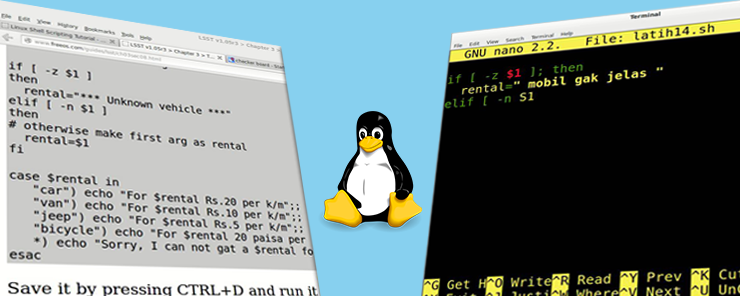In the last two articles, we have talked about the first two types of Loops: the for loop, and the while loop. Now, we are going to talk about the third and last type of loops: the until loop. After that, we will take the Loops topic to an end, by talking about Loop Control. So, here we go.

The until Loop
The until loop uses a different logic than the ones used in the former types. While the for loop iterates over a list or range of values, and the while loop iterates while a condition is true, the until loop continues to execute its loop body “until” a condition becomes true.

Syntax:

```until EXPRESSION
do
statement1
statement2
...
statementn
done
```

Where EXPRESSION is the test condition which controls whether the loop would execute one more time, or should exit.

Example
Again with the factorial example, but this time using the until loop. Check this modified version of the factorial script.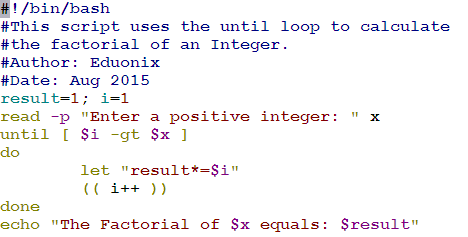Okay, let’s see it in action: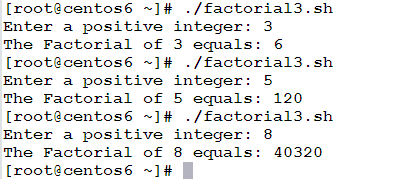Great!! Now, let’s explain it.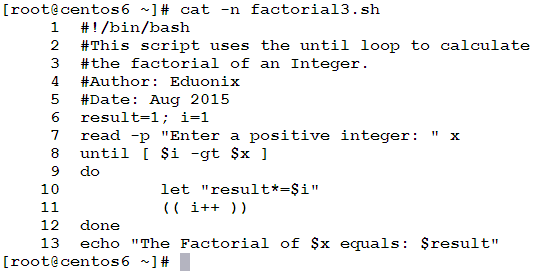• Line 6: initializes the two variables result and i  to 1.
• Line 7: uses the read command to ask the user to enter an integer, and then assigns the entered number to the variable x.
• Line 8: the until loop. The loop defines the condition for continuation: the loop will continue to execute its body until i  becomes greater than x. in other words, the loop will continue as long as i is not greater than x; i.e: i is less than or equal to x.

– i is less than or equal to x?! That sounds familiar!!

Yeah, it should be, you know why?! Because that was the condition used in the while loop (in the last article). Actually, the while and until loops are very similar in structure, and in almost everything, except only one thing: the condition. They use opposite conditions to achieve the same task. In the factorial example, the while loop used the condition [ \$i –le \$x ] which is read as: execute while i is less than or equal to x.
On the other hand, the until loop used the condition [ \$i -gt \$x ] which is read as: execute until the i becomes greater than x.
Got the difference?! Perfect!!

• The remaining lines are just copy/paste replica from the factorial2.sh script (that uses while loop), so no need to explain them.

Controlling Loops
Now, to the last section in the Loops topic: Controlling Loops. There will be some cases wherein you need to skip the execution of specific loop iteration, or to completely escape from the loop.  For these cases, Linux shell has the continue and break statements. In most cases, their execution depends on a condition.

The continue Statement
The continue statement skips the execution of the remaining lines in the current loop iteration. When seen by the interpreter, it transfers control to the next iteration.Example
Consider we need to print numbers from 1 to 100, excluding multiples of 3. i.e. print 1, 2, and skip 3, 4, 5, and skip 6, etc.
How could we achieve this?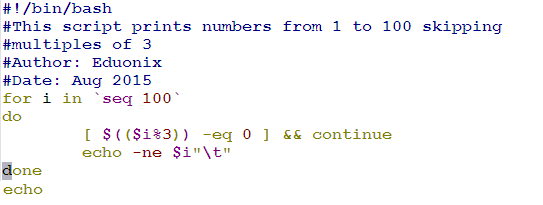Let’s see it in action.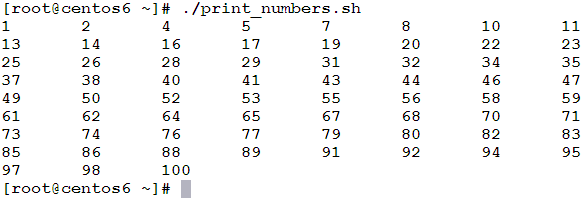Well, let’s see how this works.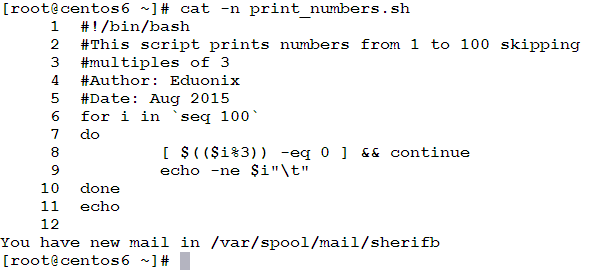• Line 6: starts a for loop with a loop variable i that iterates on the sequence of numbers from 1 to 100.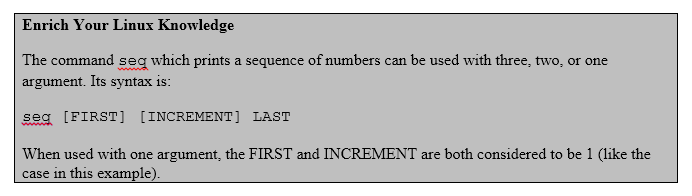• Lines 7 and 10: the do and done statements that delimit the loop body.
• Lines 8 and 9: the loop body:
• Line 8 (the star of this script): uses a test condition to check whether the reminder of dividing the number in hand (the current value of the loop variable i) by 3 is equal to 0. When the reminder of the division by 3 is zero, this means the dividend (numerator) is a multiple of 3. The test condition is followed by the logical AND “&&” operator, and then a continue statement. This makes the execution of the continue statement dependent on result of the test condition; if evaluated to true (i is a multiple of 3), the continue statement is executed, and the number i is not printed. If not, the continue statement won’t be executed, hence i will be printed.
•   Line 9: prints the current value of the loop variable i, followed by the Tab character “\t”.
• Line 11: uses the echo command without any arguments to print an empty line, to prevent the Linux shell prompt from being displayed on the same line after the script output (just after the number 100).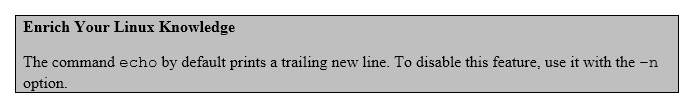The break Statement
The break statement is used when we need to exit the loop. A common usage of break statement is with infinite loops.

Example
In the following example, we are going to use the break statement to exit an infinite loop when the user enters an empty string.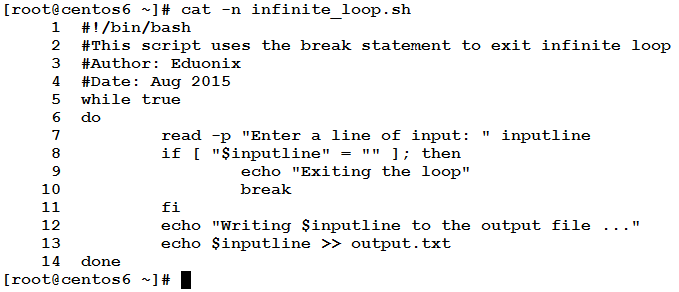Let’s see it in action.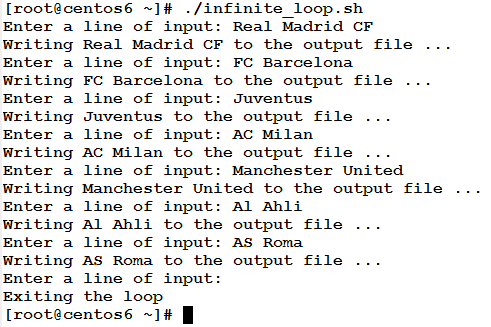Now, to the explanation.

• Line 5: while true

I know this statement looks weird, but think of it as follows: the while loop is usually followed by a test condition. And what are test conditions?! They are expressions that evaluate to either true or false. Okay, simply I substituted the condition that should evaluate to either true or false by the literal true value. So simple!! Consequently, as the while loop condition is always true (because it is literally true), the loop will never exit. This is what we call infinite loop.

• Lines 6 and 14: are the loop body delimiters
• Line 7: reads the input from the user and assigns it to the variable inputline.
• Line 8: uses if statement to check whether the input is an empty string (no input). If so, Lines 9 and 10 are executed:
• Line 9: prints a message that the loop is about to exit.
• Line 10: uses the break statement to exit the loop.
• Line 11: the closing fi for the if statement.
• Line 12 and 13 are executed if the user has entered a non-empty string input.

* * * * * *

In this article, we have talk about the third type of loops: until loop. After that, we learned how to control loops using the continue and break statements. Now, the loops topic is complete. The next article will be a new topic, so don’t miss it.